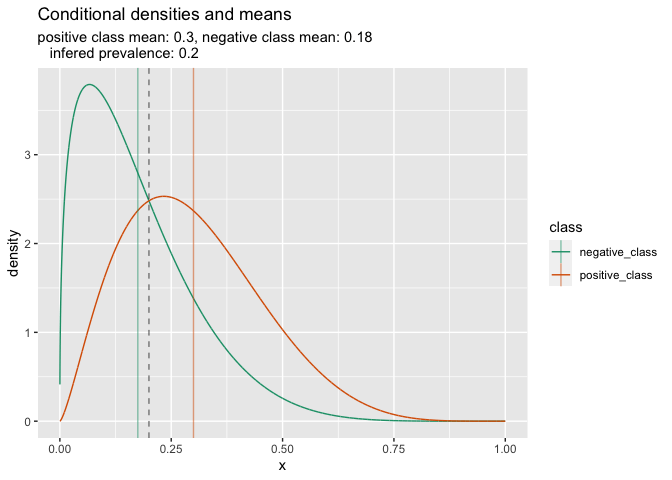# The Double Density Plot Contains a Lot of Useful Information

The double density plot contains a lot of useful information.

This is a plot that shows the distribution of a continuous model score, conditioned on the binary categorical outcome to be predicted. As with most density plots: the y-axis is an abstract quantity called density picked such that the area of each curve integrates to 1.

An example is given here.The really cool observation I wanted to share is: if we know this classifier is well calibrated, then we can recover the positive category prevalence from the graph.

A well calibrated probability score is one such that `E[outcome == TRUE] = E[prediction]`. For such a classifier we must have for the unknown positive outcome prevalence `p`. This is because the following relation holds in this case:

```   p E[prediction | on positive curve] +
(1 - p) E[prediction | on negative curve] = p
```

This follows as `p` and `1-p` are the relative sizes of the positive and negative classes, prior to being re-scaled to integrate to one as part of the density. The conditional expectations `E[prediction | on positive curve]` and `E[prediction | on negative curve]` are depicted on the double density plot, so from them we can recover the prevalence `p`.

The recovery of the prevalence from the two conditional means is shown in the earlier figure.

We have some additional results coming out for what I am currently calling “fully calibrated probability scores.” These are scores such that `E[outcome == TRUE | prediction = p] = p` for all `p` in the interval `[0, 1]`. This includes a very interesting special case where it is easy to show that the prevalence is the probability value where the density curves cross.

Tagged as:### jmount

Data Scientist and trainer at Win Vector LLC. One of the authors of Practical Data Science with R.